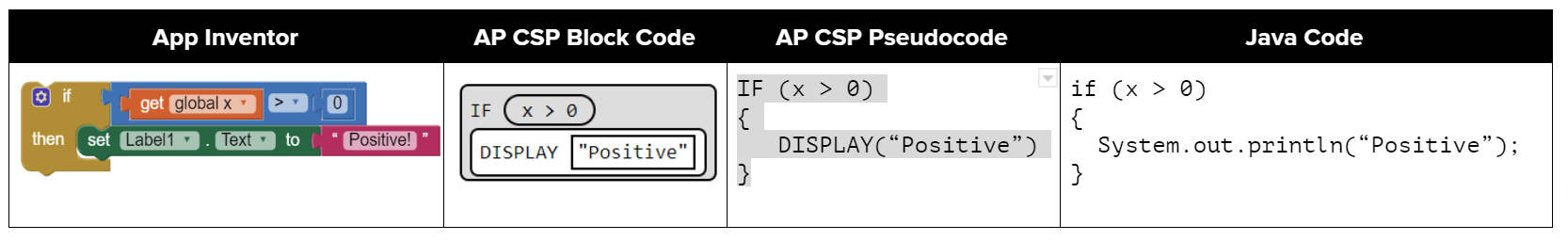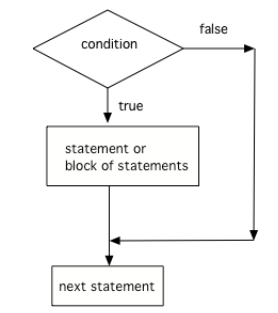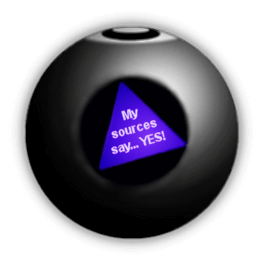# 3.2. if Statements and Control Flow¶

If you took an AP CSP course or used a block programming language like Scratch, you’ve probably seen if blocks or statements before. If statements are found in all programming languages as a way to make choices. Here’s a comparison of ifs in App Inventor blocks, AP CSP block and pseudocode and Java ifs.Figure 1: Comparison of App Inventor if block, AP CSP ifs, and Java if statements

The statements in a Java main method normally run or execute one at a time in the order they are found from top to bottom. If statements (also called conditionals or selection) change the flow of control through the program so that some code is only run when something is true. In an if statement, if the condition is true then the next statement or a block of statements will execute. If the condition is false then the next statement or block of statements is skipped.Figure 2: The order that statements execute in a conditional

A conditional uses the keyword if followed by Boolean expression inside of an open parenthesis ( and a close parenthesis ) and then followed by a single statement or block of statements. The single statement or block of statements are only executed if the condition is true. The open curly brace { and a close curly brace } are used to group a block of statements together. It is recommended to always put in the curly braces even if you have just one statement under the if statement. The questions you will see on the AP exam will use curly braces.

// A single if statement
if (boolean expression)
Do statement;
// Or a single if with {}
if (boolean expression)
{
Do statement;
}
// A block if statement: { } required
if (boolean expression)
{
Do Statement1;
Do Statement2;
...
Do StatementN;
}


Note

Note that there is no semicolon (;) at the end of the boolean expression in an if statement even if it is the end of that line. The semicolon goes at the end of the whole if statement, often on the next line. Or { } are used to mark the beginning and end of the block of code under the if condition.

Imagine that your cell phone wanted to remind you to take an umbrella if it was currently raining in your area when it detected that you were leaving the house. This type of thing is going to become more common in the future and it is an area of research called Human Computer Interaction (HCI) or Ubiquitous Computing (computers are everywhere).

The variable isRaining is a boolean variable that is either true or false. If it is true then the message Take an umbrella! will be printed and then execution will continue with the next statement which will print Drive carefully. Run the code below to see this.Check your understanding

3-2-1: Try changing the code above to boolean isRaining = false;. What will it print?

## 3.2.1. Relational Operators in If Statements¶

Most if statements have a boolean condition that uses relational operators like ==, !=, <, >, <=, >=, as we saw in the last lesson.

Run the following active code a couple times until you see all the possible outputs. It prints out whether a random number is positive or equal to 0. Add another if statement that tests if it is a negative number.

Note

A common mistake in if statements is using = instead of == in the condition by mistake. You should always use ==, not =, in the condition of an if statement to test a variable. One equal sign (=) assigns a value to a variable, and two equal signs (==) test if a variable has a certain value.Check your understanding

3-2-2: Consider the following code segment. What is printed as a result of executing the code segment?

int x = 3;
if (x > 2)
{
x = x * 2;
}
if (x > 4)
{
x = 0;
}
System.out.print(x);

• 3
• x is changed by the if statements.
• 6
• What happens when x is greater than 2 and then greater than 4? Do both if statements.
• 0
• If x is greater than 2, it's always doubled, and then that result is always greater than 4, so it's set to 0 in the second if statement.
• 4
• x is changed by the if statements.
• The code will not compile
• This code will compile.

## 3.2.2. Common Errors with If Statements¶

Here are some rules to follow with if statements to avoid some common errors:

• Always use curly brackets { and } to enclose the block of statements under the if condition. Java doesn’t care if you indent the code – it goes by the { }.

• Don’t put in a semicolon ; after the first line of the if statement, if (test);. The if statement is a multiline block of code that starts with the if condition and then { the body of the if statement }.

• Always use ==, not =, in the condition of an if statement to test a variable. One = assigns, two == tests!

The code below doesn’t work as expected. Fix it to only print “Wear a coat” and “Wear gloves” when isCold is true.

## 3.2.3.Programming Challenge : Magic 8 Ball¶Have you ever seen a Magic 8 ball? You ask it a yes-no question and then shake it to get a random response like “Signs point to yes!”, “Very doubtful”, etc. If you’ve never seen a Magic 8 ball, check out this video.

We encourage you to work in pairs for this challenge. Come up with 8 responses to yes-no questions. Write a program below that chooses a random number from 1 to 8 and then uses if statements to test the number and print out the associated random response from 1-8. If you need help with random numbers, see lesson 2.9.

Here’s a repl version that uses the Scanner class to first have the user ask a question. You can add your code in from above and try running it.

## 3.2.4. Summary¶

• if statements test a boolean expression and if it is true, go on to execute the following statement or block of statements surrounded by curly brackets { } like below.

// A single if statement
if (boolean expression)
Do statement;
// A block if statement
if (boolean expression)
{
Do Statement1;
Do Statement2;
...
Do StatementN;
}

• Java boolean expressions can compare primitive values and reference values with the relational operators == and != and arithmetic expression values with the relational operators (i.e., <, >, <=, >=).

• Conditional (if) statements affect the flow of control by executing different statements based on the value of a Boolean expression.